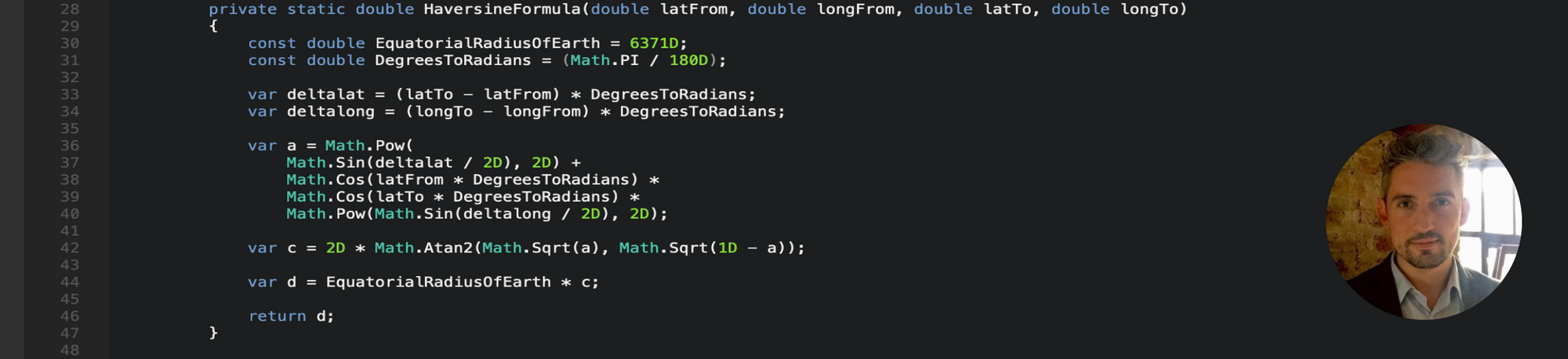February 15, 2019

# Parallel Computations of The Haversine Formula - C# .Net Core### The Spherical Distance Between Us

A common feature for modern applications is the need to supply the user with an accurate navigational distance between themselves and another endpoint, especially when providing an ETA for an item they have purchased using said piece of software.

### The Law of Haversines

Navigation is driven heavily by the law of haversines - a specific branch of spherical trigonometry which focuses on the relationships between sides and angles, particularly when it comes to polygons.

### The Haversine Formula

The haversine formula is the calculation of the great-circle distance, specifically using the latitude and longitude of two endpoints.

The great-circle distance (also known as the orthodromic distance) is the shortest distance between two points on the surface of a sphere. The key thing to note is that this distance is measured along the surface of the sphere (as opposed to a straight line directly through the sphere’s interior).

### Haversine Formula Implementation

I’ll refrain from delving into too much detail on the mathematical side of the Haversine formula. The following image shows the implementation we are going to use when running this calculation in C# .Net Core.

### Parallel Mathematical Computation

With the current hardware available in the market, we are able to utilise multi-core processors to ensure we maximise throughput and response times. Since version 4.0 of the .Net Framework, this has been made more accessible using the parallel extensions component of .Net which includes the Task Parallel Library (TPL), particularly when it involves loops as a method of execution.

The Parallel.For loop uses a ThreadPool to execute the work by invoking a delegate once per each iteration of the loop.

It’s worth highlighting that when we choose to use parallel loops, we incur a number of overhead costs -

• The cost of partitioning the source collection.
• The cost of synchronizing the worker threads.

For this example, I’ve produced a list of latitudes and longitudes that span various cities. Let’s see how the dataset is handled across a variety of different parallel computation methods!

### “Parallelism Always Beats Sequential Execution”

….and here are the results: